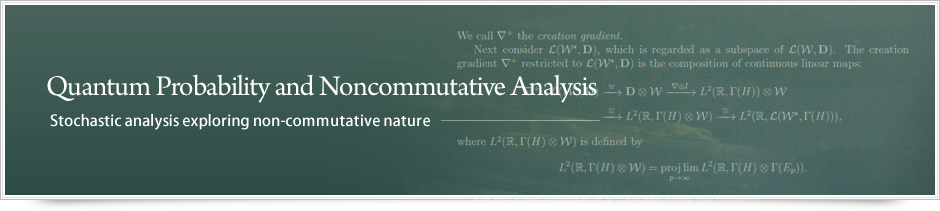##Probability theory, tracing back to the study of gambling, has developed as stochastic analysis (differential and integral calsulus in infinitely many variables) in the contemporary mathematics. The study of algebraic structure and combinatorial nature in probability theory has accelerated the progress of quantum (noncommutative) probability, exploring the origin of statistical property in noncommutativity. My research is driven by mathematical interests as well as by communication with researchers in other fields of science, for instance, network science.

2014.02.28